### Home > CALC > Chapter 6 > Lesson 6.4.2 > Problem6-140

6-140.
1. WHAT A CARD!

2. The value, V, of a Honus Wagner baseball card in mint condition appreciates at 12% per year. It was sold at auction in 1991 for \$461,000. If t = time in years since 1991, then V = 461000(1.12)t . Homework Help ✎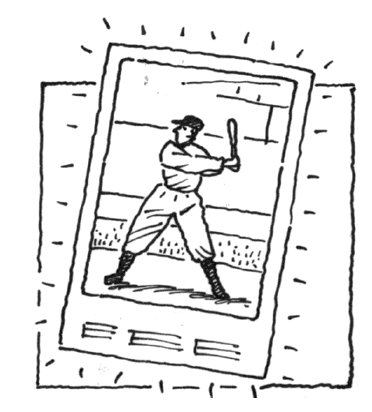1. What was its value in 2000?

2. Find the average value of the card over the period 1991 - 2000.

3. When was the card worth the value you found in part (b)?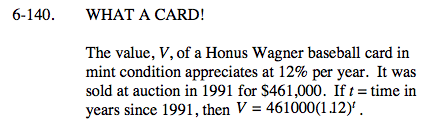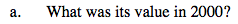We are given a value function, V(t). Notice that t = years since 1991. Evaluate for the year 2000.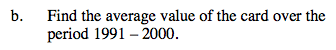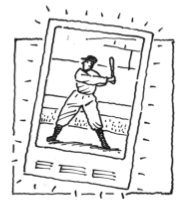Value = V(t) = 461,100(1.12)t

Examine the equation above:

What is the annual interest rate?

What is the initial value of the card?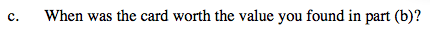Unlike average value (AROC), actual value (IROC) must be found with a derivative.